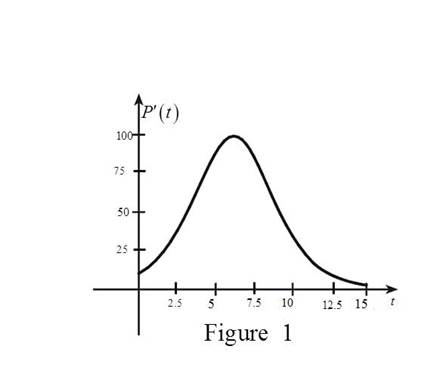# The graph of P ′ and illustrate about the yeast population.### Single Variable Calculus: Concepts...

4th Edition
James Stewart
Publisher: Cengage Learning
ISBN: 9781337687805### Single Variable Calculus: Concepts...

4th Edition
James Stewart
Publisher: Cengage Learning
ISBN: 9781337687805

#### Solutions

Chapter 2.7, Problem 12E
To determine

## To Sketch: The graph of P′ and illustrate about the yeast population.

Expert Solution

### Explanation of Solution

Given that, the graph represents the population function P(t) for yeast cells in a laboratory culture.

From the graph, it is very clear that the slope of the graph is always positive. This implies that the derivative of the graph must have a positive functional value.

Initially, the value of P(t) is very small and its starts increasing rapidly before t=5 hours and then the increase becomes negligible just after t=10 and becomes constant

after a very long time.

This implies that the graph of P(t) initially starts with a very low value and it keep increasing rapidly and then reaches the maximum value between t=5 and t=10.

Then, the value of P(t) decreases and gets closer to zero.

Graph:

Use the above information and trace the graph of P(t) as shown below in Figure 1.Illustration:

The graph indicates about the rapid growth of yeast in between t=5 and t=10 and then the growth rate declines afterwards.

### Have a homework question?

Subscribe to bartleby learn! Ask subject matter experts 30 homework questions each month. Plus, you’ll have access to millions of step-by-step textbook answers!